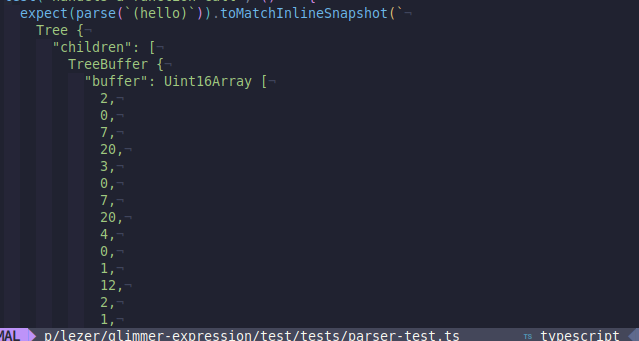# Is there a lezer API to parse to a string?

I was looking at

And i think that this format is really good for parser testing:

``````
Script(
BlockComment,
VariableDeclaration(let, Identifier, BlockComment, "=", Number))
``````

but, when using expect | Vitest
I’d like to be able to parse to the above format, so that the automatic snapshot updating has this compact syntax within it (rather than use testTree).

Today,
if I do:

``````test("handels a function call", () => {
expect(parser.parse(`(hello)`)).toMatchInlineSnapshot()
});
``````

after the test suite runs, I get a structure like this:which makes sense, because the `tree` object isn’t meant for human’s it’s meant for the editor / codemirror stuff.

but for testing, I’d like to have some sort of “blessed” toString conversion of that that looks more like the expected syntax in the docs.

Something like this:

``````expect(parser.parse('(hello)').humanReadable()).toEqual(`
Script(
BlockComment,
VariableDeclaration(let, Identifier, BlockComment, "=", Number))
`)
``````

I see that pars of this behavior is provided by the `TestSpec` here: generator/src/test.ts at main · lezer-parser/generator · GitHub
(though this is the inverse – converting the human-friendly syntax to something compareable to a tree)

I’m still poking through the lezer repos / code – so apologies if this already exists.

lol, it’s just `tree.toString()`

Good job, me

I have this helper:

``````import { parser } from "@glimdown/lezer-glimmer-expression";

export function parse(input: string) {
let tree = parser.parse(input);

return tree.toString();
}
``````

and usage:

``````import { describe, test, expect } from "vitest";

import { parse } from "./util";

test("handels a function call", () => {
expect(parse(`(hello)`)).toMatchInlineSnapshot(
'"Expression(SExpression(CallExpression(\\"(\\",SExpression,\\")\\")))"'
);
});
``````

where the argument to match inline snapshot is:

• filled if missing (by vitest)
• diffed / asserted if present

Diff could be better:

``````- Expected

- "Expression(SExpression(CallExpression(\"(\",SExpression,\")\")))"
+ "Expression(SExpression(CallExpression(\"(\",SExperession,\")\")))"
``````

But maybe there is way to also parse the toString() and add newlines / indentation (this part I can do just fine)

Here is my formatter now,
end:tm: result:

``````import { describe, test, expect } from "vitest";

import { parse } from "./util";

test("handels a function call", () => {
expect(parse(`(hello)`)).toMatchInlineSnapshot(`
"Expression(
SExpression(
CallExpression(
\\"(\\",SExpression,\\")\\"
)
)
)"
`);

expect(parse(`(hello "there")`)).toMatchInlineSnapshot(`
"Expression(
SExpression(
CallExpression(
\\"(\\",⚠
)
)  ,SExpression(
String(
AttributeValueContent
)
)  ,⚠(
\\")\\"
)
)"
`);
});

``````

and the test util:

``````import { parser } from "@glimdown/lezer-glimmer-expression";

export function parse(input: string) {
let tree = parser.parse(input);

let stringifiedTree = tree.toString();

return format(stringifiedTree);
}

function format(flatTree) {
let result = "";
let indent = 0;
let inQuote = false;

let quoteSplit = flatTree.split(/("[^"]+")/g);

let sections = quoteSplit
.map((s) => {
if (s.startsWith('"') && s.endsWith('"')) {
return s;
}

return s.split(/([()])/g);
})
.flat()
.filter(Boolean);

// Combine groups of non ( or )
let combined = sections.reduce((acc, current) => {
let last = acc[acc.length - 1];

if (last) {
if (!last.endsWith("(") && last !== ")") {
if (current !== "(" && current !== ")") {
acc[acc.length - 1] = last + current;

return acc;
}
}

if (!last.endsWith("(") && current === "(") {
acc[acc.length - 1] = last + current;

return acc;
}
}

acc.push(current);

return acc;
}, []);

for (let section of combined) {
if (section.endsWith("(")) {
if (inQuote) break;
result += indentFor(indent) + section + "\n";
indent += 2;
} else if (section.endsWith(")")) {
if (inQuote) break;
indent -= 2;
result += "\n" + indentFor(indent) + section;
} else {
result += indentFor(indent) + section;
}
}

return result;
}

function indentFor(size = 0) {
return Array(size).fill(" ").join("");
}
``````

Still needs some formatting work.

But what I like about this workflow is that I can spot check the tests / diffs as I go and just decide to delete all the snapshots if I need to change things, and the test framework will fill in the “expected” for me